X
items
Product Qty Amount
 From: Until:

Archive
[email protected]
/ Categories: Archive, fasteners

# Back to Basics - Part 2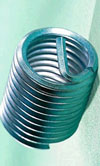In the previous article, we looked at the relationship between tightening torque and axial force developed, and a formula was given which included the effects of those parameters which, as engine designers, you are most likely to want to change. In this article, we shall look at the effects of cyclic loading – it is necessary to understand this in order to determine the proper pre-load. This process of doing fatigue calculations and checking that the pre-load is sufficient is an iterative process, and may involve changing materials, fastener geometry

, joint geometry or manufacturing methods. There is some very simple maths involved, and the units I use are SI, so apologies to our readers who use ‘English’ units. However, we all use the same version of physics, so there should be nothing to puzzle anybody.

We need to have a good understanding of the joint behaviour as it becomes loaded, in order to determine the loads in the fastener. First, let us imagine that the bolt has been tightened until the joint is just held together, i.e. there is no actual tension in the bolt, and no compression in the joint. If a force then acts to separate the joint, the whole of this force is taken by the bolt, whose behaviour in this situation is identical to that if it had been tensioned in isolation.

This situation changes dramatically when the joint is pre-loaded by tightening the bolt further before the joint is subjected to working loads. In this situation, we must consider the elastic behaviour of the bolt and the joint and treat them as simple elastic components, springs if you like. We might consider the bolt to have a stiffness k1, and the joint to have stiffness k2. If we imagine that these are concentric and that we impose a deflection on the two springs, we can see that the force developed depends on the familiar equation

F = kx

where:
F is the force in Newtons
k is the stiffness in Newtons per metre
x is the deflection in the joint

In this case:

F = k1x + k2x, or F = x(k1 + k2)

The part of the total force used which increases the load in the bolt F1 = k1x

We can therefore define a ‘load coefficient’ which will determines the cyclic loads seen by the fastener.

F = F1 / F, i.e. F = k1 / (k1 + k2)

where:
F is the load coefficient, which is dimensionless
F1 is the force in the bolt in Newtons
F is the total force in Newtons
k1 is the stiffness of the bolt in Newtons per metre
k2 is the stiffness of the joint components in Newtons per metre

From this simple equation, we can draw a very useful fact. We can say that the smaller the load coefficient, the smaller the proportion of cyclic load seen by the fastener. This can help to steer us in the right direction for materials choice, bolt geometry and joint geometry.

So, if we say that the bolt is tightened to a preload Fp, and that the joint is cyclically loaded in tension by a force Fc, we can determine the maximum and minimum loads in the fastener and, with further information, construct a Goodman diagram to satisfy ourselves that the parts should not fail by fatigue.

Fmin = Fp
Fmax = Fp + FFc

where
Fmin is the minimum force in the bolt in Newtons
Fmax is the minimum force in the bolt in Newtons

So, if we preload the bolt by 20 units of force, subject the joint to a further 20 units of force as a working load we assume and the bolt has a stiffness one-ninth of that of the joint, i.e. k2 = 9k1, we can calculate the load co-efficient, and loads in the fastener and joint:

F = k1 / (k1 + k2) = k1 / (k1 + 9k1) = 1/10 = 0.1

So, when the joint is tensioned by the 10 units of force, the bolt sees one-tenth of this, and the force at the joint face is relieved by nine-tenths of this.

For the bolt:

Fmin = 20
Fmax = 22

For the joint:
Fmax = 20
Fmin = 2

We can see that, despite the fact that we have added an extra 20 units of force to the joint (which was equal to the preload in the bolt), there are two conclusions which might surprise the newcomer to bolt calculations:

there remains some force clamping the joint together
the maximum force in the bolt is only increased slightly

These are important conclusions and we shall build on this in further articles on the subject of bolted joints and fasteners.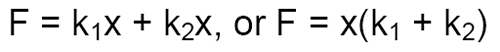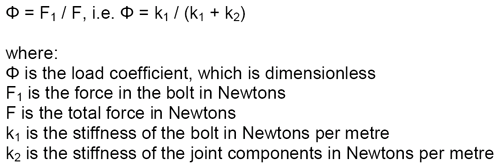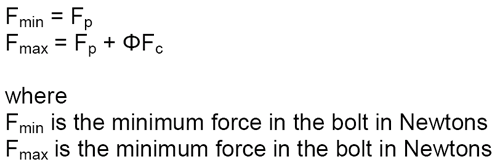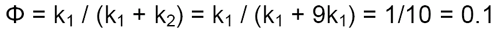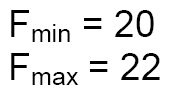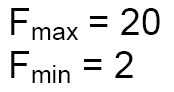Written by Wayne Ward.

Previous Article Protect and Survive
Next Article Exhaust Materials